## Introduction to Pandas Histograms

Histograms are a powerful tool for analyzing the distribution of data. Plots like histograms that characterize the distribution of individual variables or features are vital for data analysis because they help reveal patterns in the input data. Pandas DataFrames that contain our data come pre-equipped with methods for creating histograms, making preparation and presentation easy.

We can create histograms from Pandas DataFrames using the `pandas.DataFrame.hist` DataFrame method, which is a sub-method of `pandas.DataFrame.plot`. Pandas uses the Python module Matplotlib to create and render all plots, and each plotting method from `pandas.DataFrame.plot` takes optional arguments that are passed to the Matplotlib functions. In this tutorial, we will cover the essential tools and options for plotting histograms from Pandas DataFrames, but you should be aware that many more options (e.g. plot color, orientation, size, etc.) are available to be passed to the Matplotlib via `pandas.DataFrame.plot.hist`.

If you’re trying to find out how to plot your Pandas DataFrame data in a histogram, we’re going to assume you’ve already built your Pandas DataFrame. We’ve already built ours, too! To illustrate creating histograms, we’re going assume we have the following DataFrame df containing test grades already available within our Python environment:

``````df.info()
> <class 'pandas.core.frame.DataFrame'>
> RangeIndex: 746 entries, 0 to 745
> Data columns (total 3 columns):
> Test_1    746 non-null float64
> Test_2    746 non-null float64
> Type      746 non-null int64
> dtypes: float64(2), int64(1)
> memory usage: 17.6 KB

>        Test_1     Test_2  Type
> 0   85.868553  88.160763     2
> 1   88.017580  89.946783     3
> 2   76.811083  82.444485     3
> 3  100.000000  68.406203     3
> 4   94.719547  63.994755     2``````

I put together a Python Developer Kit with over 100 pre-built Python scripts covering data structures, Pandas, NumPy, Seaborn, machine learning, file processing, web scraping and a whole lot more - and I want you to have it for free. Enter your email address below and I'll send a copy your way.

Yes, I'll take a free Python Developer Kit

Here we have two features, Test_1 and Test_2, along with a group number for each test given by the feature Type. A “feature” is just a what we call a piece of measurable information about a topic.

If we would like to create a fast and simple histogram for exploratory analysis, we can use the simple `pandas.DataFrame.hist` 1 method, like this:

``df.hist()``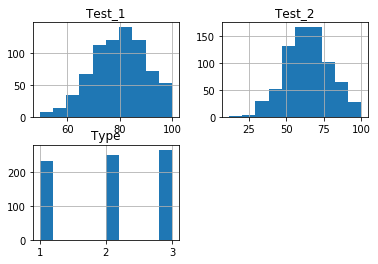This command produced histograms for each of the 3 features we specified. However, if we want to perform additional analysis by altering the plots, we will have to specify additional options when calling our histograms.

## Single Histogram from a Pandas DataFrame

The simple `df.hist()` method above plotted histograms of every feature in the DataFrame. If we wish to only examine a subset of the features, or even look at only one, then we can specify what we want to plot using the `columns` parameter of the `df.hist()` method. The `columns` feature takes either a string or list of strings of columns names:

``df.hist(column="Test_1")  # Plot a single column``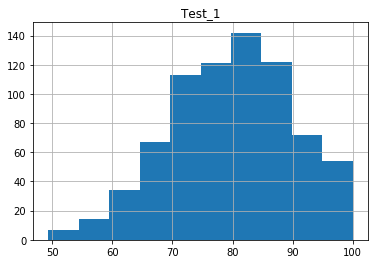We’ll demonstrating plotting multiple histograms with a list of strings shortly.

### Modifying Histogram Bin Sizes

The bins, or bars of the histogram plot, can be adjusted using the `bins` option. This option can be tuned depending on the data, to see either subtle distribution trends, or broader phenomena. Which bin size to use heavily depends on the data set you use, therefore it is important to know how to change this parameter. The default number of bars is 10.

``df.hist(column="Test_1", bins=5)  # Plotting 5 bins``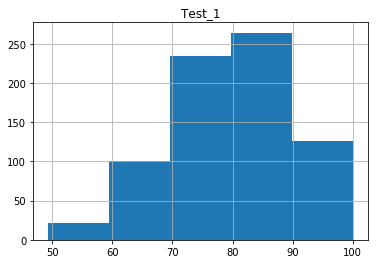``df.hist(column="Test_1", bins=30)  # Plotting 30 bins``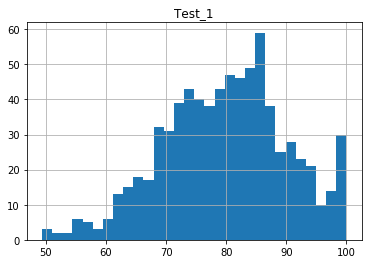## Multiple Pandas Histograms from a DataFrame

The `columns` feature can take a list of column names to produce separate plots for each chosen column:

``df.hist(column=["Test_1", "Test_2"])  # Plot specific columns``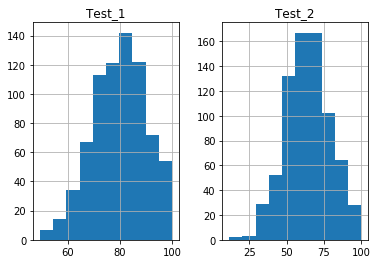The histograms will be plotted side-by-side for you. Notice the axes are automatically adjusted by default, so the scales may be different for each Pandas DataFrame histogram.

### Modifying Histogram Axes

Again, you may notice in the above plots, the x and y axes are not the same. Different scales can complicate side-by-side data comparisons, so we would prefer to set both of the axes to the same range and scale. We can do this with the `sharex` and `sharey` options. These options accept boolean values, which are False by default. If these options are set to True, then the respective axis range and scale is shared between plots:

``df.hist(column=["Test_1", "Test_2"], sharex=True)  # Share only x axis``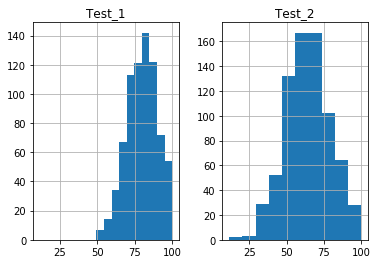``df.hist(column=["Test_1", "Test_2"], sharex=True, sharey=True)  # Share x and y axis``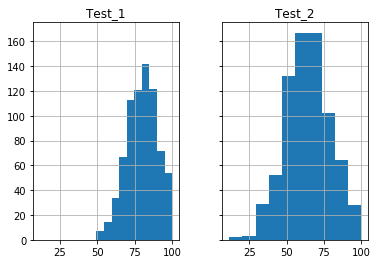Note: Be careful when comparing histograms this way. The range over which bins are set in the Test_1 data are smaller than those in the Test_2 data, leading to larger boxes in Test_2 than Test_1. The result is that while both plots have the same number of data points,Test_2 appears “larger” because of the default bar widths.

### Plotting Multiple Features in One Plot

Suppose we wanted to present the histograms on the same plot in different colors. To do this, we will have to slightly change our syntax and use the `pandas.DataFrame.plot.hist` method. This `plot.hist` method contains more specific options for plotting. It does not, however, contain a `columns` option, therefore we will have to slice the DataFrame prior to calling the method.

Recall our DataFrame had 3 columns of data. To only plot the Test_1 and Test_2 data, we’ll need to slice it like this:

``df[["Test_1", "Test_2"]].plot.hist()  # Note slicing is performed on df itself``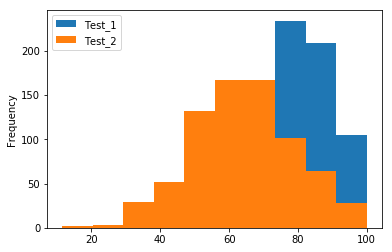This code snippet plotted both histograms on the same plot, but the second plot “blocks” the view of the first. We can solve this problem by adjusting the `alpha` transparency option, which takes a value in the range `[0,1]`, where 0 is fully transparent and 1 is fully opaque.

``df[["Test_1", "Test_2"]].plot.hist(alpha=0.4)  # Plot at 40% opacity``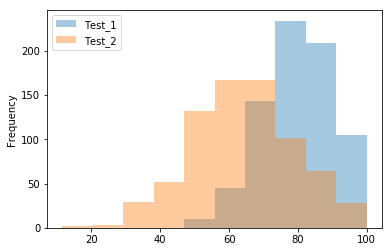Now we can see the intersection of the plots. It’s worth noting that not all the plotting features are available in the Pandas plotting methods. Be aware that if the Pandas methods do not have the options you wish to apply to your plots, you’ll have to resort to using manual Matplotlib functions.

### Grouping Pandas data with 'by' Option

We’re going to end this tutorial by talking about something a little more complicated. Suppose our data is grouped according to some feature of the data, or that our data exists in categories. In this case, we may want to determine the distributions of each type separately. In our example data, these categories are specified by the Type column, which is the third column in our DataFrame.

We can create multiple plots of data grouped by a feature using the `by` option of the `pandas.DataFrame.hist` method. The `by` option will take an object by which the data can be grouped. This can include a string of the column name you use to separate the data. This is exactly what we’re doing in our final example:

``df.hist(by="Type", column="Test_1")``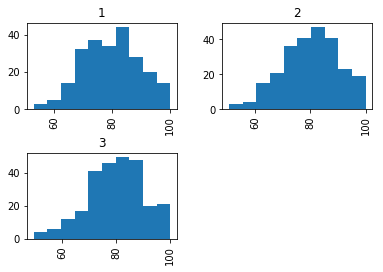Histograms are just one way of plotting the distribution of data. For another helpful method, check out our tutorial on creating Pandas Density Plots, which plot the normalized frequency of occurrences in our dataframe.

On a related note, you can also plot a normalized histogram by setting the `normed` argument to True, like this:

``df["Test_1"].plot.hist(normed=True)``

1. Notice that this fast method of histogram creation is the method `pandas.DataFrame.hist`, rather than `pandas.DataFrame.plot.hist` (with the `plot` method explicitly included). The `pandas.DataFrame.hist` method contains default settings that are more applicable to fast, though simple, exploratory analysis. The `pandas.DataFrame.plot.hist` will be used when we wish to specify more options for plotting. See the Manual Page for additional information.